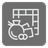Square Board
Elementary+
English RU

You are designing a board game in which tokens move around the square board with. (like in Monopoly (Wikipedia))

You need to write the function that returns the coordinates after the token has moved. The coordinates of a token are being represented by a tuple consisting of a row and column. The coordinates of a top-left one - (0, 0).

You are given three arguments:
• the first one is the length of the game board’s side (an integer);
• the second is the token’s current position (an integer);
• Cells around the board are numbered clockwise starting with 0 on the bottom right corner.

• the third is the number of steps to advance (an integer);
• A positive number represents a clockwise direction. A negative number represents a counterclockwise direction.

Example:

```square_board(4, 1, 4) == (1, 0)
square_board(6, 2, -3) == (4, 5)
```Input: 3 arguments: the length of the game board’s side (an integer), token’s current position (an integer), and the number of steps to advance (an integer).

Output: The coordinates after the token has moved (a tuple consisting of a row and column).

How it is used:
To get the coordinates of an object that moves around.

Precondition:

• 3 ≤ The length of the side ≤ 11
• 0 ≤ The position of the token < (side-1)*4